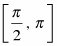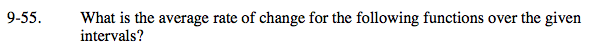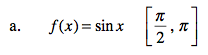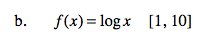### Home > AC > Chapter 16 > Lesson 16.9.1.4 > Problem9-55

9-55.
1. What is the average rate of change for the following functions over the given intervals? Homework Help ✎

1. f(x) = sin x2. f(x) = log x [1, 10]$\frac{\sin(\pi) - \sin\left(\frac{\pi}{2}\right)}{\pi - \frac{\pi}{2}} = ?$$\frac{f(10) - f(1)}{10 - 1} = ?$

log(1) = 0